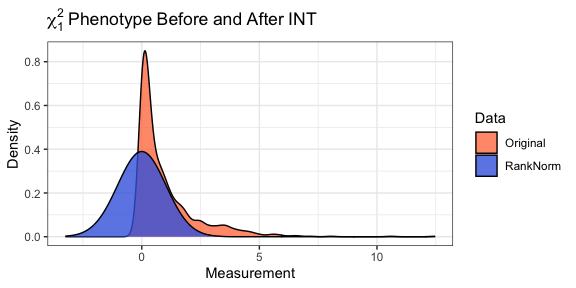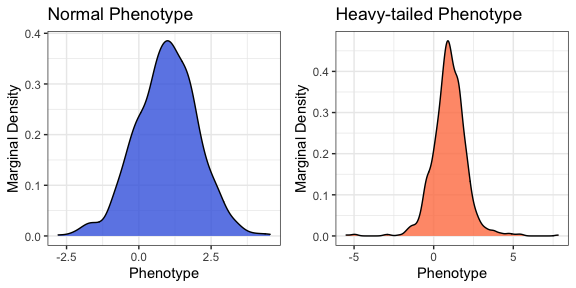# Setting

Consider genetic association analysis with a continuous trait. If the residual distribution is asymmetric (skewed) or heavy-tailed (kurtotic) relative to the normal distribution, then standard linear regression may fail to control the type I error in moderate samples. Even if the sample is sufficiently large for standard linear regression to provide valid inference, it is not fully efficient when the residual distribution is non-normal. Examples of traits that may exhibit non-normal residual distributions include body mass index, circulating metabolites, gene expression, polysomnography signals, and spirometry measurements. In such cases, the rank based inverse normal transformation (INT) has been used to counteract departures from normality. During INT, the sample measurements are first mapped to the probability scale, by replacing the observed values with fractional ranks, then transformed into Z-scores using the probit function. In the following example, a sample of size $$n = 1000$$ is drawn from the $$\chi_{1}^{2}$$ distribution. After transformation, the empirical distribution of the measurements in is indistinguishable from standard normal.

library(RNOmni)

# Sample from the chi-1 square distribution.
y <- rchisq(n = 1000, df = 1)

# Rank-normalize.
z <- RankNorm(y)# Data

## Simulated Data

Here, data are simulated for $$n = 10^{3}$$ subjects. Genotypes are drawn for $$10^{3}$$ loci in linkage equilibrium with minor allele frequency between 0.05 and 0.50. The model matrix X contains an intercept, four standard normal covariates Z, and the first four genetic principal components. The intercept is set to one, and the remaining regression coefficients are simulated as random effects. The proportion of phenotypic variation explained by covariates is 20%, while the proportion of variation explained by principal components is 5%. Two phenotypes with additive residuals are simulated. The first y_norm has standard normal residuals, while the second y_tail has $$t_{3}$$ residuals, scaled to have unit variance.

set.seed(100)

# Sample size.
n <- 1e3

# Simulate genotypes.
maf <- runif(n = 1e3, min = 0.05, max = 0.50)
G <- sapply(maf, function(x) {rbinom(n = n, size = 2, prob = x)})
storage.mode(G) <- "numeric"
G <- scale(G)

# Genetic principal components.
pcs <- svd(G, nu = 4, nv = 0)\$u[,1:4]

# Covariates.
Z <- matrix(rnorm(n * 4), nrow = n)
Z <- scale(Z)

# Overall design matrix.
X <- cbind(1, Z, pcs)

# Coefficients.
b <- c(
1,
rnorm(n = 4, sd = 1/sqrt(15)),
rnorm(n = 4, sd = 1/sqrt(60))
)

# Linear predictor.
h <- as.numeric(X %*% b)

# Normal phenotype.
y_norm <- h + rnorm(n)

# Heavy-tailed phenotype.
y_tail <- h + rt(n, df = 3) / sqrt(3)## Data Formatting

The outcome y is expected as a numeric vector. Genotypes G are expected as a numeric matrix, with subjects are rows, and variants as columns. If adjusting for covariates or population structure, X is expected as a numeric matrix, which should contain an intercept. Factors and interactions should be expanded in advance, e.g. using model.matrix. Missingness is not permitted in either the outcome vector y or the model matrix X, however the genotype matrix G may contain missing values. Observations with missing genotypes are excluded from association testing only at those loci where the genotype is missing.

# Basic Association Test

The basic association test is linear regression of the (untransformed) phenotype on genotype and covariates. BAT provides an efficient implementation using phenotype y, genotypes G, and model matrix X. Standard output includes the score statistic, its standard error, the Z-score, and a p-value, with one row per column of G. Setting test = "Wald" specifies a Wald test. The Wald test may provide more power, but is generally slower

# Basic Association Test, Normal Phenotype
bat_y_norm <- BAT(y = y_norm, G = G, X = X)
cat("BAT Applied to Normal Phenotype:\n")
cat("\n\n")

# Basic Association Test, Heavy-tailed Phenotype
cat("BAT Applied to Heavy-tailed Phenotype:\n")
bat_y_tail <- BAT(y = y_tail, G = G, X = X)
round(head(bat_y_tail), digits = 3)
## BAT Applied to Normal Phenotype:
##
## X1    Score     SE      Z     P
##   1  28.119 32.043  0.878 0.380
##   2 -14.633 32.240 -0.454 0.650
##   3  25.638 32.285  0.794 0.427
##   4 -38.067 32.319 -1.178 0.239
##   5  60.793 31.876  1.907 0.056
##   6 -15.410 31.994 -0.482 0.630
##
##
## BAT Applied to Heavy-tailed Phenotype:
##
## X1    Score     SE      Z     P
##   1  16.664 32.860  0.507 0.612
##   2 -64.387 33.062 -1.947 0.051
##   3  24.400 33.109  0.737 0.461
##   4 -16.016 33.143 -0.483 0.629
##   5  56.952 32.689  1.742 0.081
##   6 -12.206 32.811 -0.372 0.710

# Inverse Normal Transformation

Suppose that a continuous measurement $$u_{i}$$ is observed for each of $$n$$ subjects. Let $$\text{rank}(u_{i})$$ denote the sample rank of $$u_{i}$$ when the measurements are placed in ascending order. The rank based inverse normal transformation (INT) is defined as:

$\text{INT}(u_{i}) = \Phi^{-1}\left\{\frac{\text{rank}(u_{i})-k}{n-2k+1}\right\}.$

Here $$\Phi^{-1}$$ is the probit function, and $$k\in(0,1/2)$$ is an adjustable offset. By default, the Blom offset of $$k = 3/8$$ is adopted.

# Direct INT

In direct INT (D-INT), the INT-transformed phenotype is regressed on genotype and covariates. D-INT is most powerful when the phenotype could have arisen from a rank-preserving transformation of a latent normal trait. DINT implements the association test using phenotype y, genotypes G, and model matrix X. Standard output includes the test statistic, its standard error, the Z-score, and a p-value, with one row per column of G. Wald and score tests are available.

# Direct INT Test, Normal Phenotype.
cat("D-INT Applied to Normal Phenotype:\n")
dint_y_norm <- DINT(y = y_norm, G = G, X = X)
cat("\n\n")

# Direct INT Test, Heavy-tailed Phenotype.
cat("D-INT Applied to Heavy-tailed Phenotype:\n")
dint_y_tail <- DINT(y = y_tail, G = G, X = X)
round(head(dint_y_tail), digits = 3)
## D-INT Applied to Normal Phenotype:
##
## X1    Score     SE      Z     P
##   1  30.544 33.944  0.900 0.368
##   2 -12.230 34.153 -0.358 0.720
##   3  26.580 34.201  0.777 0.437
##   4 -36.773 34.237 -1.074 0.283
##   5  62.961 33.767  1.865 0.062
##   6 -16.303 33.893 -0.481 0.631
##
##
## D-INT Applied to Heavy-tailed Phenotype:
##
## X1    Score     SE      Z     P
##   1   8.917 35.725  0.250 0.803
##   2 -70.214 35.944 -1.953 0.051
##   3  12.055 35.995  0.335 0.738
##   4 -24.941 36.033 -0.692 0.489
##   5  62.189 35.539  1.750 0.080
##   6 -15.625 35.671 -0.438 0.661

# Indirect INT

In indirect INT (I-INT), the phenotype and genotypes are first regressed on covariates to obtain residuals. The phenotypic residuals are rank normalized. Next, the INT-transformed phenotypic residuals are regressed on genotypic residuals. I-INT is most powerful when the phenotype is linear in covariates, but the residual distribution is skewed or kurtotic. IINT implements the association test, using phenotype y, genotypes G, and model matrix X. Standard output includes the test statistic, its standard error, the Z-score, and a p-value, with one row per column of G.

# Indirect INT Test, Normal Phenotype.
cat("I-INT Applied to Normal Phenotype:\n")
iint_y_norm <- IINT(y = y_norm, G = G, X = X)
cat("\n\n")

# Indirect INT Test, Heavy-tailed Phenotype.
cat("I-INT Applied to Heavy-tailed Phenotype:\n")
iint_y_tail <- IINT(y = y_tail, G = G, X = X)
round(head(iint_y_tail), digits = 3)
## I-INT Applied to Normal Phenotype:
##
## X1    Score     SE      Z     P
##   1  27.391 31.204  0.878 0.380
##   2 -15.266 31.395 -0.486 0.627
##   3  25.637 31.439  0.815 0.415
##   4 -37.707 31.473 -1.198 0.231
##   5  57.583 31.041  1.855 0.064
##   6 -16.402 31.156 -0.526 0.599
##
##
## I-INT Applied to Heavy-tailed Phenotype:
##
## X1    Score     SE      Z     P
##   1  12.867 31.204  0.412 0.680
##   2 -52.078 31.395 -1.659 0.097
##   3  16.650 31.439  0.530 0.596
##   4 -13.190 31.473 -0.419 0.675
##   5  54.267 31.041  1.748 0.080
##   6 -12.796 31.156 -0.411 0.681

# Omnibus INT

Since neither D-INT nor I-INT is uniformly most powerful, the INT omnibus test (O-INT) adaptively combines them into a single robust and statistically powerful test. Internally, OINT applies both D-INT and I-INT. The corresponding p-values are transformed into Cauchy random deviates, which are averaged to form the omnibus statistic. In general the omnibus p-value will be between the D-INT and I-INT p-values, but closer to smaller of these two. OINT implements the omnibus test, using phenotype y, genotypes G, and model matrix X. The standard output includes the p-values estimated by each of D-INT, I-INT, and O-INT.

# Omnibus INT Test, Normal Phenotype.
cat("O-INT Applied to Normal Phenotype:\n")
oint_y_norm <- OINT(y = y_norm, G = G, X = X)
cat("\n\n")

# Omnibus INT Test, Heavy-tailed Phenotype.
cat("O-INT Applied to Heavy-tailed Phenotype:\n")
oint_y_tail <- OINT(y = y_tail, G = G, X = X)
round(head(oint_y_tail), digits = 3)
## O-INT Applied to Normal Phenotype:
##   DINT-p IINT-p OINT-p
## 1  0.368  0.380  0.374
## 2  0.720  0.627  0.678
## 3  0.437  0.415  0.426
## 4  0.283  0.231  0.255
## 5  0.062  0.064  0.063
## 6  0.631  0.599  0.615
##
##
## O-INT Applied to Heavy-tailed Phenotype:
##   DINT-p IINT-p OINT-p
## 1  0.803  0.680  0.753
## 2  0.051  0.097  0.067
## 3  0.738  0.596  0.676
## 4  0.489  0.675  0.590
## 5  0.080  0.080  0.080
## 6  0.661  0.681  0.672